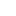• ### 几个模糊集空间的性质

分类： 数学 >> 数学（综合） 提交时间： 2022-06-30Huan Huang

摘要：This paper discusses the properties the spaces of fuzzy sets in a metric space?equipped with the endograph metric and the sendograph metric, respectively.?We fist give some relations among the endograph metric, the sendograph?metric and the Γ-convergence, and then investigate the level characterizations?of the endograph metric and the Γ-convergence. By using the above results,?we give some relations among the endograph metric, the sendograph metric,?the supremum metric and the dp* metric. On the basis of the above results,?we present the characterizations of total boundedness, relative compactness?and compactness in the space of compact positive α-cuts fuzzy sets equipped?with the endograph metric, and in the space of compact support fuzzy sets?equipped with the sendograph metric, respectively. Furthermore, we give?completions of these metric spaces, respectively.

同行评议状态:待评议

• ### 具有Lp度量的模糊集空间的性质

分类： 数学 >> 数学（综合） 提交时间： 2022-06-20黄欢

In this paper, we discuss the properties of  the spaces of fuzzy sets in a metric space with $L_p$-type $d_p$ metrics, $p\geq 1$. Firstly, we give the characterizations of compactness in fuzzy set space with $d_p$ metrics. Then we present the completions of fuzzy set spaces with $d_p$ metrics.
The $d_p$ metrics are well-defined if and only if a function induced by the Hausdorff metric is measurable. In this paper, we give some fundamental conclusions on the measurability of this function. This paper was submitted to Fuzzy Sets and Systems on 2022.05.06.

同行评议状态:待评议

• ### 关于豪斯多夫度量的一些讨论

分类： 数学 >> 数学（综合） 提交时间： 2022-04-30黄欢

In this paper, we discuss the properties of functions generated using Hausdorff metric.

同行评议状态:待评议

• ### Properties of fuzzy set

分类： 数学 >> 数学（综合） 提交时间： 2021-08-10黄欢

摘要：In this paper, we give some properties related to platform points of a fuzzy set and their applications.

同行评议状态:待评议